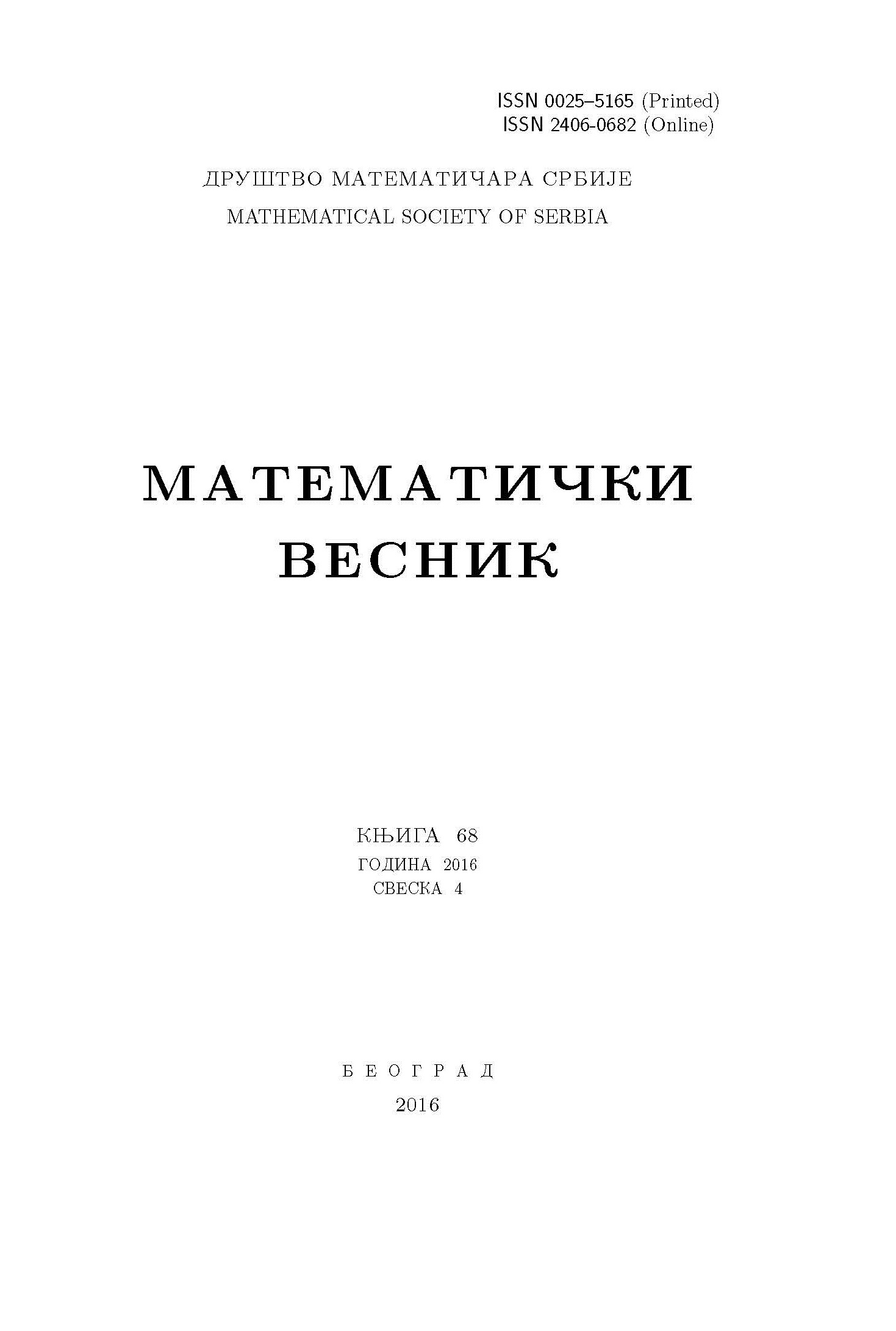﻿ Matematički Vesnik ﻿
 MATEMATIČKI VESNIK МАТЕМАТИЧКИ ВЕСНИКTWO-WEIGHTED INEQUALITIES FOR RIESZ POTENTIAL AND ITS COMMUTATORS IN GENERALIZED WEIGHTED MORREY SPACES C. Aykol, J. J. Hasanov, Z. V. Safarov AbstractIn this paper we find the conditions for the boundedness of Riesz potential $I^{\alpha}$ and its commutators from the generalized weighted Morrey spaces $\mathcal{M}^{p,\varphi_1}_{\omega_1}(\mathbb{R}^n)$ to the generalized weighted Morrey spaces $\mathcal{M}^{q,\varphi_2}_{\omega_2}(\mathbb{R}^n)$, where $0<\alphaKeywords: Maximal operator; Riesz potential; commutator; weighted Lebegue space; generalized weighted Morrey space; BMO space. MSC: 42B20, 42B25, 42B35 Pages: 37$-\$49 Volume  75 ,  Issue  1 ,  2023

﻿